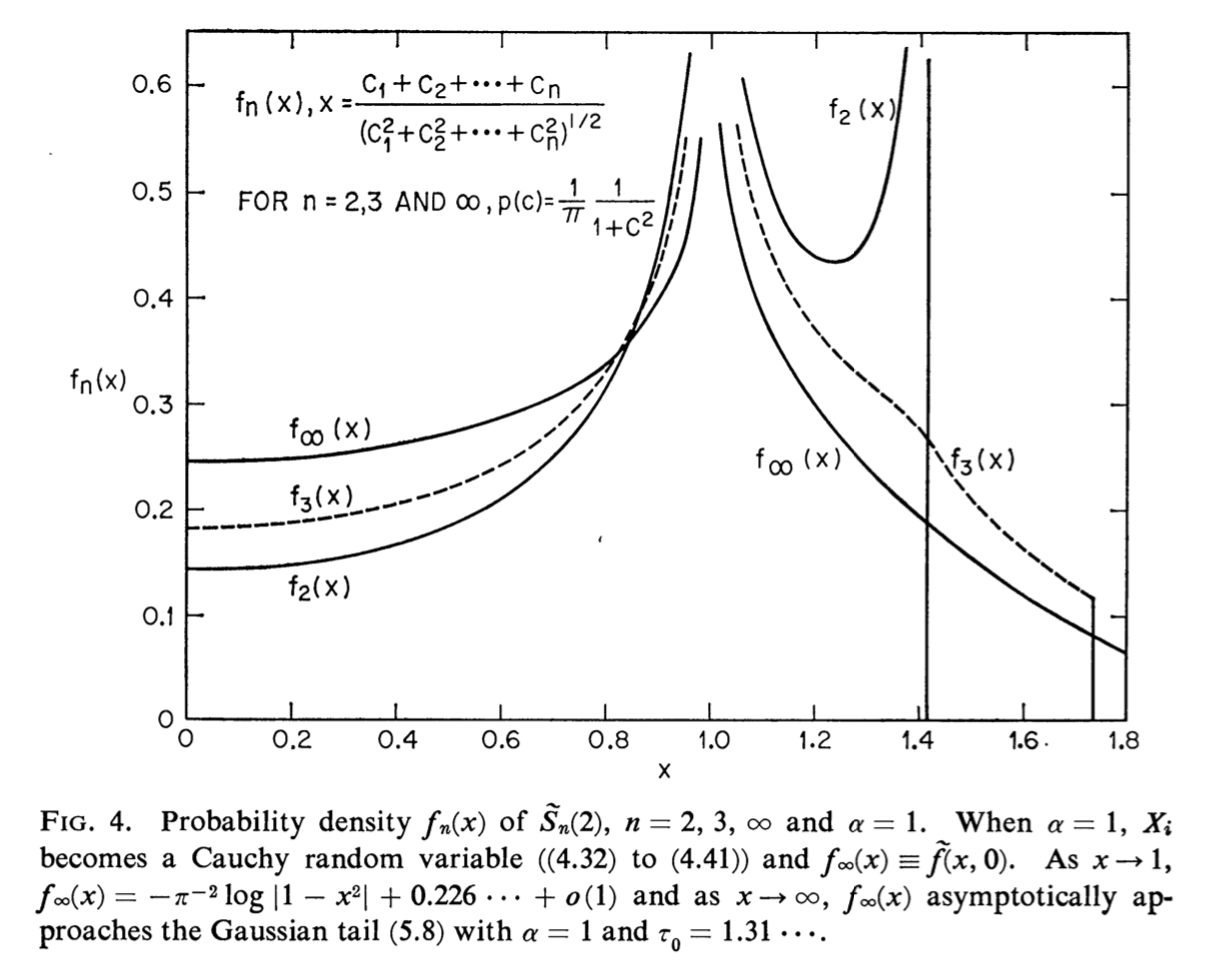# Large-n limit of the distribution of the normalized sum of Cauchy random variables

What is the large-n limit of a distribution of the following sample statistic:$$x\equiv\displaystyle\frac{\sum^{n}X_{i}}{\,\sqrt{\,\sum^{n}X_{i}^{2}\,}\,}$$ when sampling the Cauchy(0,1) distribution? Monte Carlo simulation indicates that convergence to this limit is quite fast, and that the resulting (symmetric) PDF has very sharp cusps at -1 and +1, but of course does not yield an analytic expression for this PDF - would anyone be able to find it?
Here is how the positive half of the PDF looks like:• A somewhat random remark: the limiting distribution is also the distribution of $X_1 / \langle X\rangle_1$ if $X_t$ is the Cauchy process and $\langle X\rangle_t$ is the quadratic variation process, a $\tfrac{1}{2}$-stable subordinator. I bet someone has studied the joint law of $X_t$ and $\langle X\rangle_t$, but unfortunately I do not have time now to search for the reference. – Mateusz Kwaśnicki Jun 16 at 20:17

This desired large-$$n$$ limit of the distribution $$P_n(x)$$ is calculated in Limit Distributions of Self-normalized Sums (1973). The Cauchy distribution is the case $$\alpha=1$$ on page 798. The singularity in $$\lim_{n\rightarrow\infty}P_n(x)$$ at $$x=\pm 1$$ is logarithmic $$P_n(x)\rightarrow-\pi^{-2}\log|1-x^2|,$$ see equation (5.12) and figure 4 (reproduced below), while for large $$|x|$$ the decay is Gaussian.